News

# TFG6900 series signal generator function introduction

Aug. 25, 2021

Signal generator is the most basic and popular electronic measurement instrument, which is widely used in the field of wireless communication, but also in production, scientific research, national defense and scientific experiments. Signal generator is also called signal source, and its output signal type can be sine wave signal, function signal, pulse signal, arbitrary waveform signal or digital modulated signal, etc. Next, we introduce the specific functions of TFG6900 series signal.

As a signal generator, the most important function is to generate signals. The following pictures show the different waveforms generated by TFG6900 series signal generators.

First is the standard waveform.

Sine waveform: the peak-to-peak value is 20 V, the frequency is 1.5 KHz, and the offset is 0.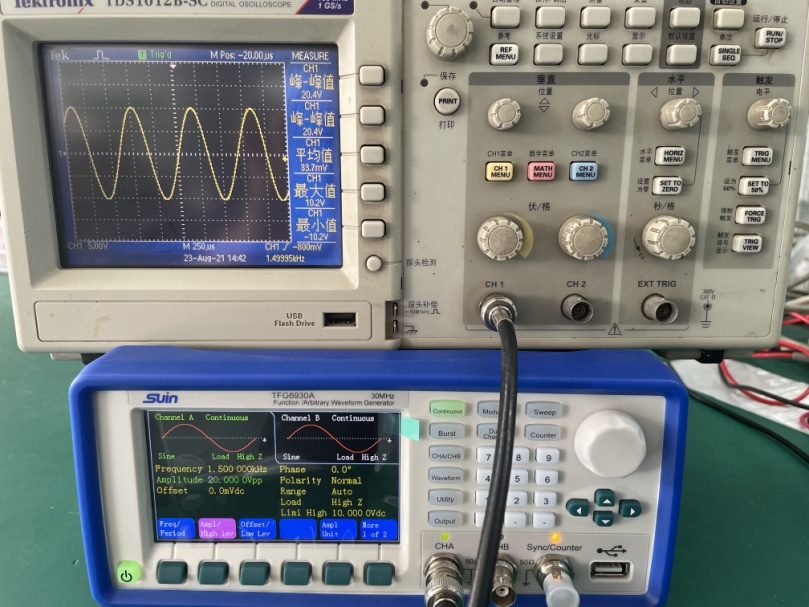Square waveform: the peak-to-peak value is 15 V, the frequency is 2.5 KHz, and the offset is 0.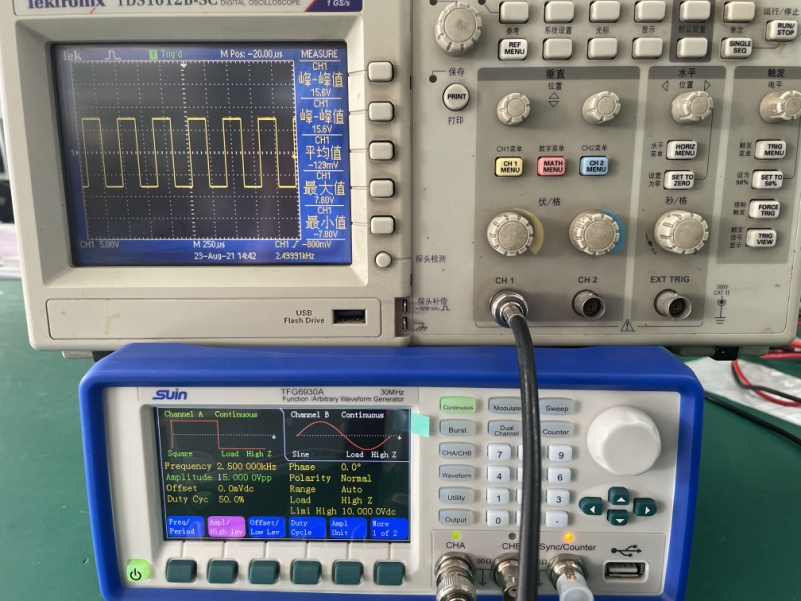Ramp waveform: peak-to-peak value is 16.5V, frequency is 2.0KHz, offset is 0.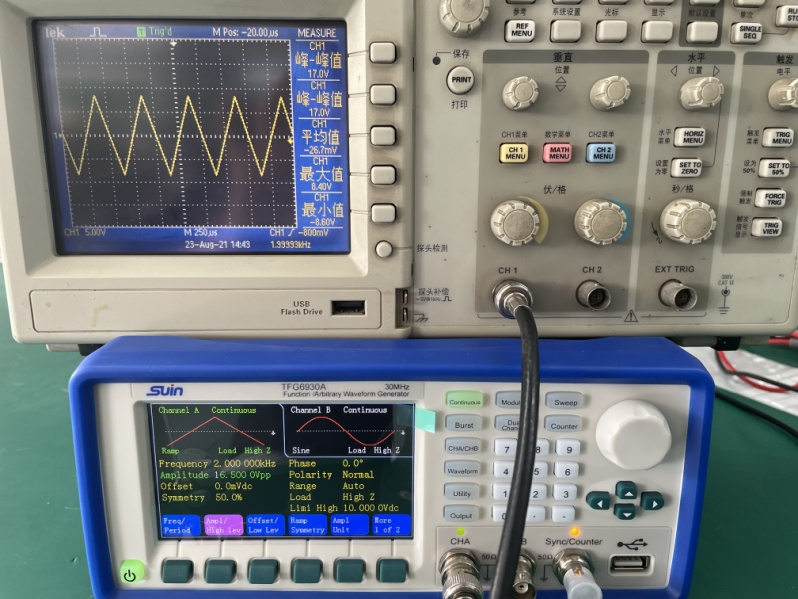Pulse wave: peak-to-peak value of 15V, frequency of 1.5KHz, offset of 0.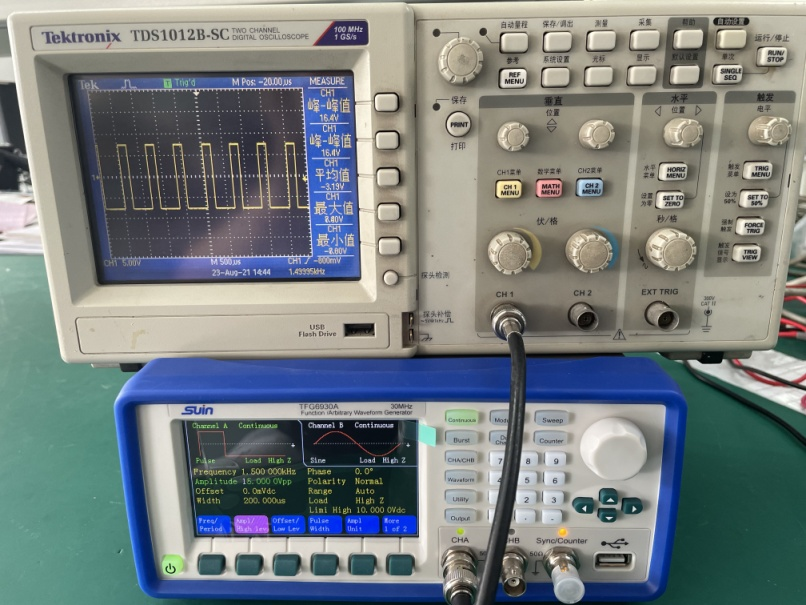In addition to the commonly used standards, the device can also generate arbitrary waveforms. The device supports 50 types of arbitrary waveforms, including exponential function, logarithmic function, tangent function, Gaussian function, pseudo-random code, ECG waveform, and vibration waveform.

In order to meet the needs of some customers for special waveforms, we can also output user-defined waveforms,that is, 5 arbitrary waveforms can be edited and stored.

Another highlight of this product is its rich modulation methods, FM, AM, PM, PWM, etc.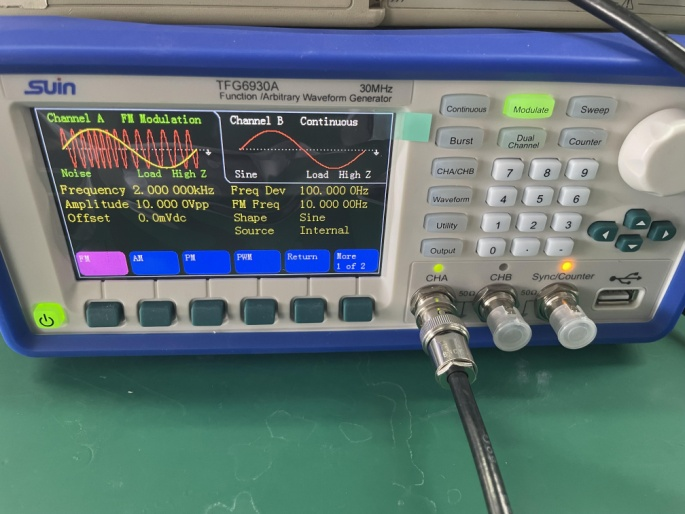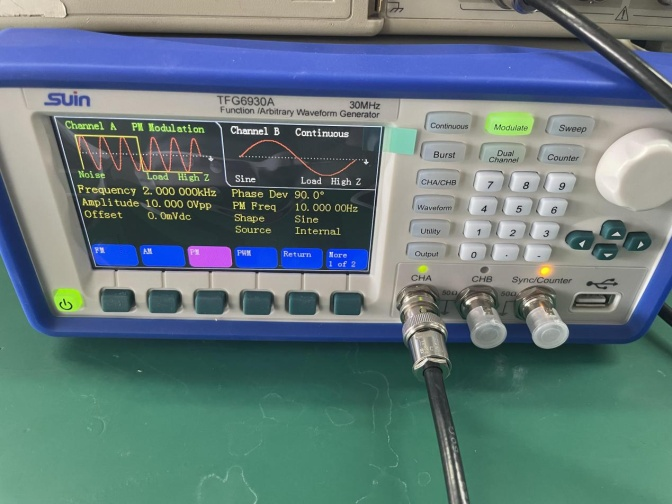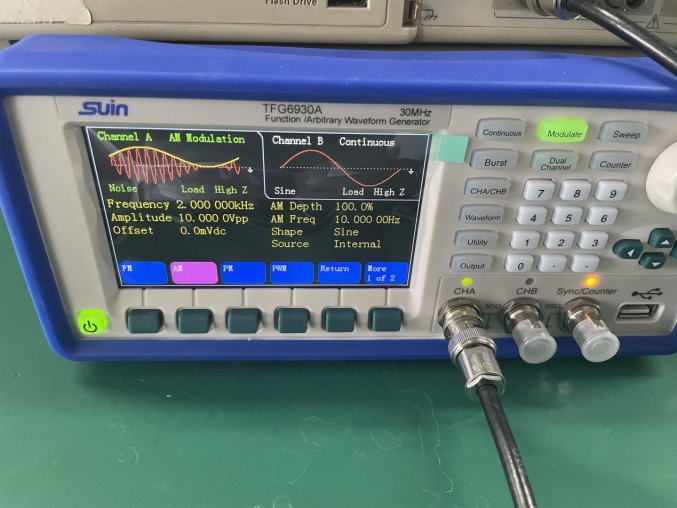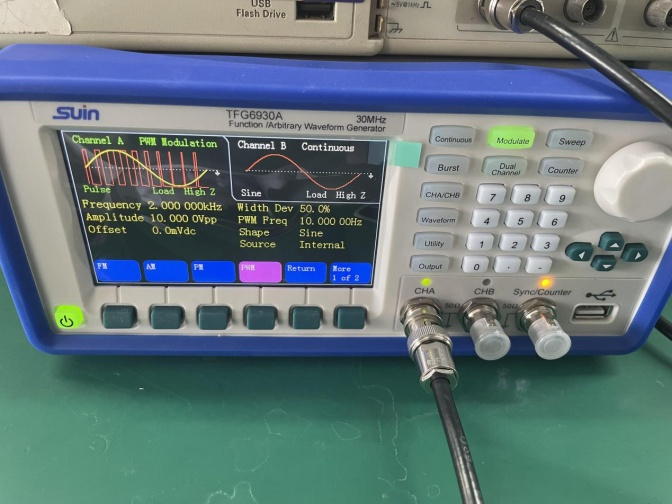All of the above are standard on this model, but customers can also install options such as a power amplifier.